## MP Board Class 9th Maths Solutions Chapter 1 Number Systems Ex 1.4

Question 1.
Visualise 3.765 on the number line, using successive magnification.
Solution:
Visualizing 3.765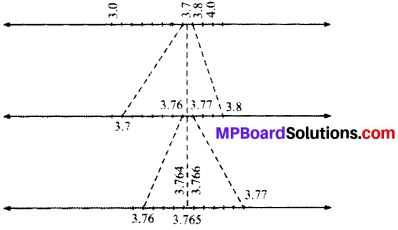Question 2.
Visualise 4.26 on the number line, up to 4 decimal places.
Solution:
Visualising 4.26 = 4.2626
= 4.263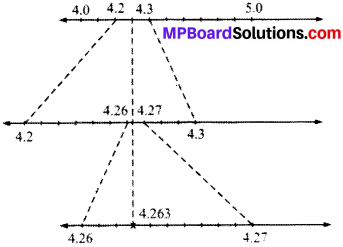Rationalisation of the Denominator of an irrational number having two terms in the Denominator:
To rationalise the denominator of an irrational number having two terms in the denominator, multiply the numerator and denominator of the number by the conjugate of its denominator.

Example 1.
If a and b are rational numbers and $$\frac { 3+\sqrt { 7 } }{ 3-\sqrt { 7 } }$$ = a + b√7 find value of a and b.
Solution:
We have $$\frac { 3+\sqrt { 7 } }{ 3-\sqrt { 7 } }$$ = a + b√7
Multiply denominator and numerator by 3 + √7 on LHS, then we have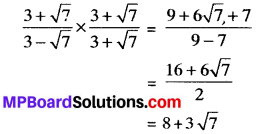On comparing LHS and RHS, we get
a = 8, b = 3

Example 2.
Determine a and b if $$\frac { 5+\sqrt { 3 } }{ 7-4\sqrt { 3 } }$$ = 94a + 3 √3b
Multiply numerator and denominator by 7 + 4√3 on LHS, then we have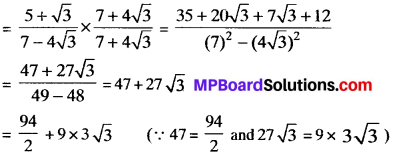On comparing LHS and RHS, we get
a = $$\frac{1}{2}$$ and b = 9.Example 3.
Determine a and 6 if $$\frac { 7+\sqrt { 5 } }{ 7-\sqrt { 5 } }$$ – $$\frac { 7-\sqrt { 5 } }{ 7+\sqrt { 5 } }$$ = a + 7√5b.
Solution:
We have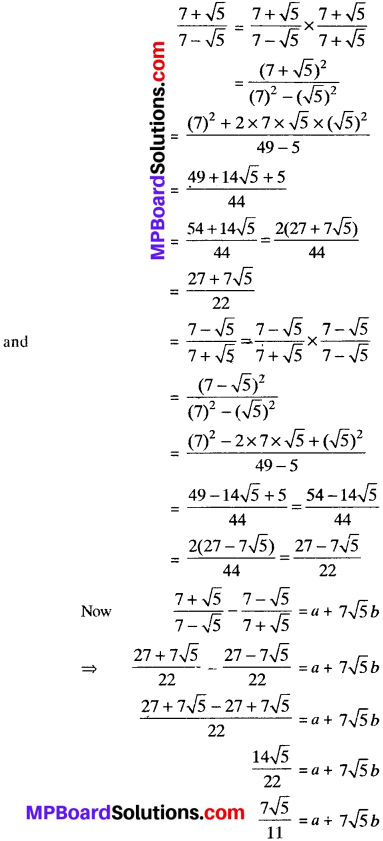On comparing LHS and RHS, we get
a = 0 and b = $$\frac{1}{11}$$.